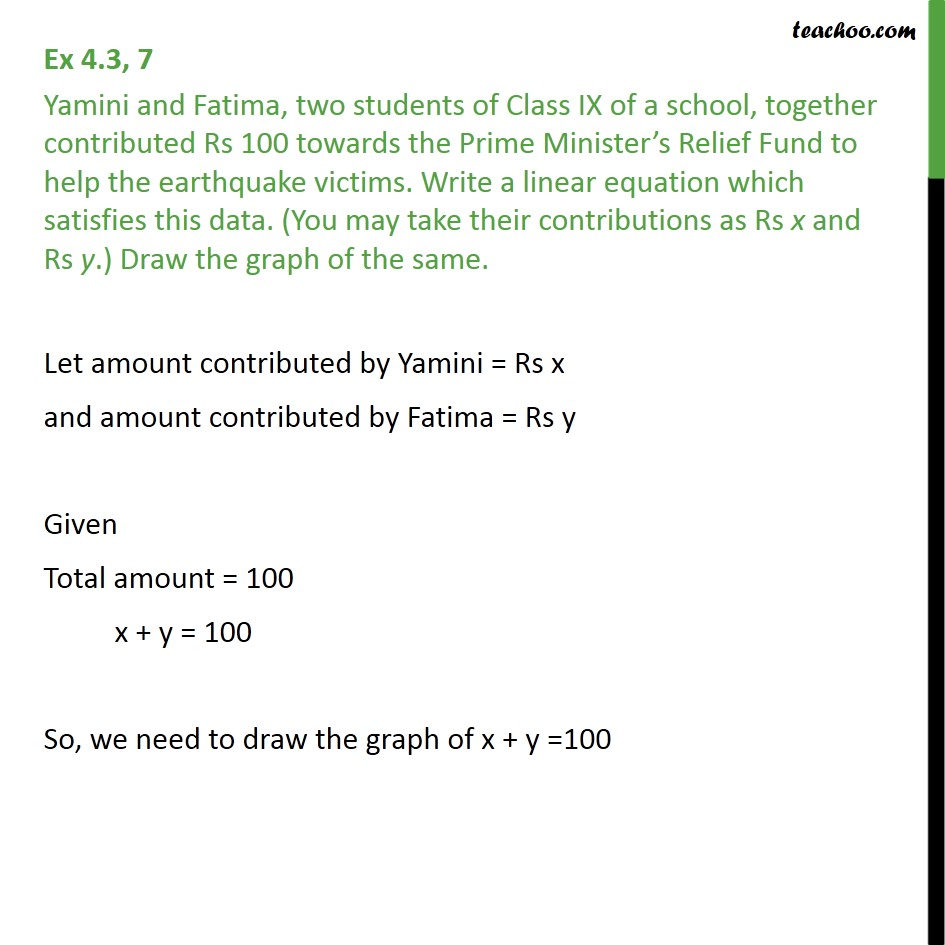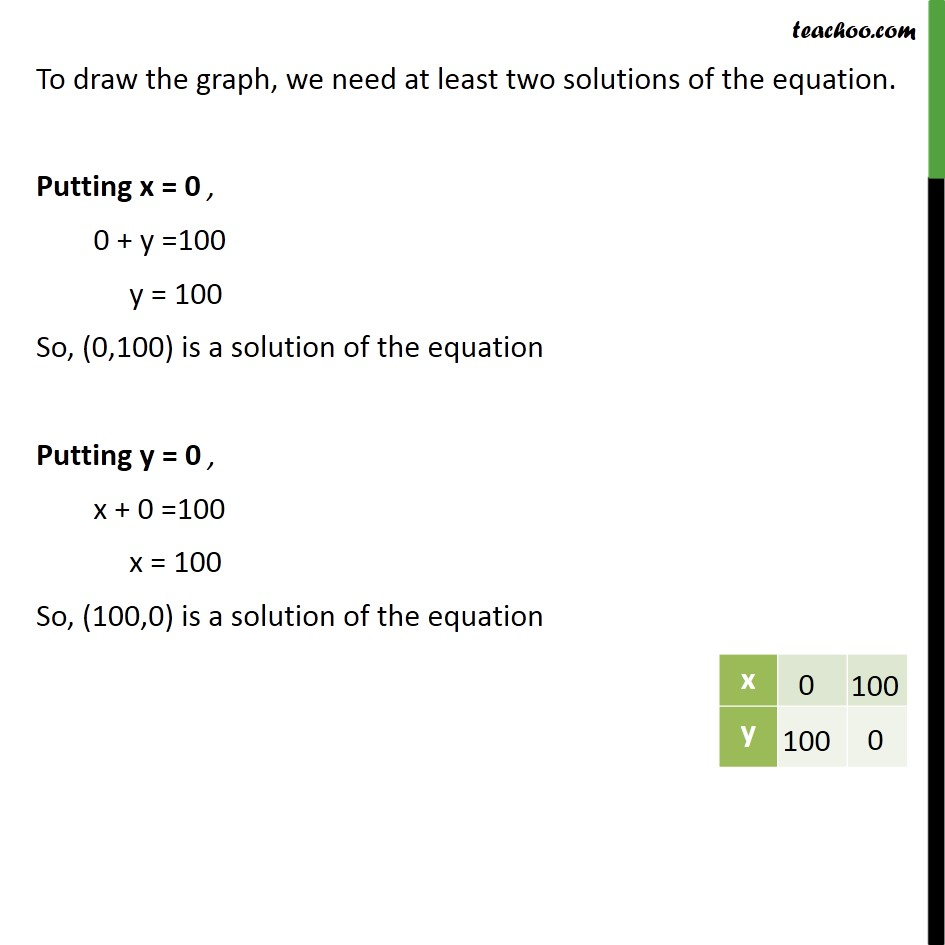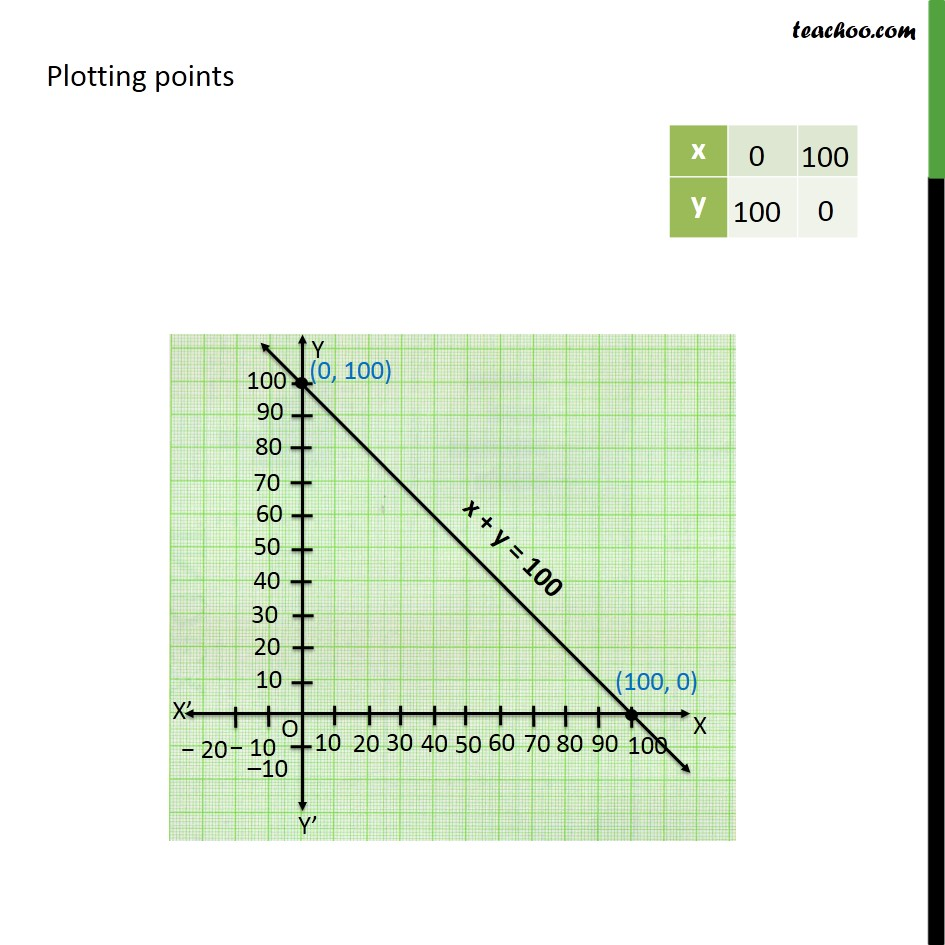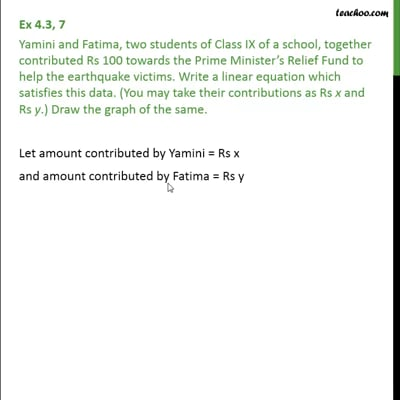Forming Equations and drawing graph

Chapter 4 Class 9 Linear Equations in Two Variables
Concept wiseThis video is only available for Teachoo black users

Introducing your new favourite teacher - Teachoo Black, at only ₹83 per month

### Transcript

Ex 4.3, 7 Yamini and Fatima, two students of Class IX of a school, together contributed Rs 100 towards the Prime Minister’s Relief Fund to help the earthquake victims. Write a linear equation which satisfies this data. (You may take their contributions as Rs x and Rs y.) Draw the graph of the same. Let amount contributed by Yamini = Rs x and amount contributed by Fatima = Rs y Given Total amount = 100 x + y = 100 So, we need to draw the graph of x + y =100 To draw the graph, we need at least two solutions of the equation. Putting x = 0 , 0 + y =100 y = 100 So, (0,100) is a solution of the equation Putting y = 0 , x + 0 =100 x = 100 So, (100,0) is a solution of the equation Plotting points